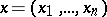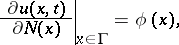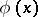# Second boundary value problem

(diff) ← Older revision | Latest revision (diff) | Newer revision → (diff)

One of the boundary value problems (cf. Boundary value problem, partial differential equations) for partial differential equations. For example, let there be given a second-order elliptic equation(*)where,, in a bounded domain, with a normal at each point of the boundary. The second boundary value problem for equation (*) inis the following problem: Out of the set of all solutions of equation (*), isolate those solutions which have, at all boundary points, derivatives with respect to the interior normaland which satisfy the conditionwhereis a given function. The second boundary value problem is also known as the Neumann problem.

How to Cite This Entry:
Second boundary value problem. Encyclopedia of Mathematics. URL: http://encyclopediaofmath.org/index.php?title=Second_boundary_value_problem&oldid=12888
This article was adapted from an original article by A.K. Gushchin (originator), which appeared in Encyclopedia of Mathematics - ISBN 1402006098. See original article